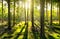# Random Forest

Understanding the forest.Let the forest make the decision
• please go through my article on Decision Trees if you are not familiar with its concepts.

Random Forest algorithm, as the name suggests, is a collection of different uncorrelated decision trees. The algorithm basically works on collective conscience.

• Classification use case: For each input all the trees vote and the class with highest number of votes wins (democracy).
• Regression use case: Usually mean of the result of all the trees is the output of the forest.

# How different uncorrelated trees are created?

This happens in two steps:

• Firstly, different datasets are created from original dataset (replacement of rows is allowed). …

# Decision Trees

How a tree decides?

What is a decision tree?

“A decision tree is a flowchart-like structure in which each internal node represents a “test” on an attribute (e.g. whether a coin flip comes up heads or tails), each branch represents the outcome of the test, and each leaf node represents a class label (decision taken after computing all attributes). The paths from root to leaf represent classification rules.” — Wikipedia

Entropy: It is the amount of information needed to accurately describe a sample. Its value lies in between 0 (homogenous) and 1 (heterogenous).

# Logistic Regression

Binary Classification.

Logistic Regression is the simplest supervised binary classification model. Binary classification implies that our target variable is dichotomous i.e. it can take two values like 0 or 1.

The Sigmoid function:

# Linear Regression

Simple & effective…

Linear Regression can be defined as modelling a line which illustrates the relationship between the response and explanatory variables.

• Response : The variable we are trying to predict. (Continuous Variable)
• Explanatory Variable(s) : The input variables in the regression analysis.

# Assumptions of Linear Regression:

• The explanatory variables should be independent and uncorrelated to each other.
• The error terms are uncorrelated with each other.
• The error term has constant variance.

# When to use Linear Regression?

• Graphical Analysis : If the graph between the label and inputs shows a linear nature.
• Technical Indicator : If the Pearson correlation coefficient between input and output variable is near -1 or…

# Python Pandas-II

Learn efficiently

If you have not read, the Pandas-Starter post in the series, here is the link.

A large chunk of a data scientist’s work revolves around data transformation. Pandas library provides various functions to transform data, aggregate data, pivot data etc. Thus making life of data scientists easier.

Let us have a look at the data we will be using as example in this post.

`import pandas as pddata = pd.read_csv("cm31MAR2021bhav.csv")data = data.drop('Unnamed: 13',axis=1)print("\n",data.columns)print("\n",data.shape)print("\n",data.head())`

# Python - Flask

Host your own API.

Flask is a web application framework. It is sometimes also called a micro framework as it keeps API core very simple.

This post will mainly focus on the application of Flask framework. Here is the link to Flask documentation if you wish to know more.

I will be using Postman to test the APIs. To know more about Postman click here.

1. Let us start with an “hello world” example of a function in Python:
`def hello_world():   return "Hello World"`

We can access the function over a network using Flask:

`from flask import Flaskapp = Flask(__name__)@app.route('/')def hello_world()…`

# Data Analytics- Introduction

What is it ?

“Data analytics is all about gaining insights from data and using it to solve a problem statement.”

It has become a part and parcel of various domains such as biological research, cosmic exploration, stock market analysis, financial management, travel planning, advertisement industry, manufacturing industry etc.

Types of data analysis:

a) Descriptive Data Analysis (What?): The amount of data under study can be humongous and to understand the aspects of it can become overwhelming. Descriptive statistics helps us in creating simple summaries which further combined with graphs and charts can be used to construct a coherent representation…

# Python- Pandas Starter

Code effortlessly.

Pandas is a python library which is mainly used for tabular data operations. It is an important part of data science toolkit. The APIs in Pandas provide a wide range of functionality and they are simple to implement.

Pandas can be installed via pip:

`pip install pandas`

The two main components of pandas library are ‘series’ and ‘dataframe’. Series can be thought of as a column in a table where dataframe is the table.

## Let us try to create a dataframe from scratch:

`import pandas as pddc = {    "col1": [1,2,3,4,5],    "col2": [2,3,4,5,6]}df = pd.DataFrame(dc)print(df)`

If we check the datatype of the ‘df’…

# Python- Numpy Starter

Code effortlessly.

Numpy stands for Numerical Python. It is the library most suited if one wishes to work with matrices, arrays, transformations etc. Its simplicity and highly efficient algorithms have made numpy a must learn library.

Let us conduct an experiment to see how numpy performs over pythons ‘for loop’ :

In the below experiment we are trying to add corresponding elements in two arrays, i.e. 1st element of one array to 1st element of second array and likewise. At the end we can compare the time taken to complete the task.

`from datetime import datetimea = [2,3,4]*100000000b = [2,4,4]*100000000start…`

# Python Basic -playing with classes

Code effortlessly

A python class gives us structure for creating a new entity in python with methods associated with it.

Default data types in python such string, list etc. all are classes (check builtins.py).

Let us look go through some examples to clear our understanding:

1. Creating a class
`class A:    age = 4        def get_age(self):        print(A.age)`

In the above example we have defined a class A and associated variable ‘age’ and method ‘get_age’ to it. Notice inside get_age method we are calling value of age as A.age which is because of the scope of variable.

`# Create an instance of…`